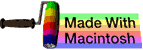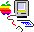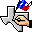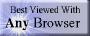music# division

## summary

This subchapter looks at division.

## free computer programming text book project

If you like the idea of this project,### stub section

This subchapter is a stub section. It will be filled in with instructional material later. For now it serves the purpose of a place holder for the order of instruction.

Professors are invited to give feedback on both the proposed contents and the propsed order of this text book. Send commentary to Milo, PO Box 1361, Tustin, California, 92781, USA.

# division

This subchapter looks at division.

## Stanford C essentials

Stanford CS Education Library This [the following section until marked as end of Stanford University items] is document #101, Essential C, in the Stanford CS Education Library. This and other educational materials are available for free at http://cslibrary.stanford.edu/. This article is free to be used, reproduced, excerpted, retransmitted, or sold so long as this notice is clearly reproduced at its beginning. Copyright 1996-2003, Nick Parlante, nick.parlante@cs.stanford.edu.

### Mathematical Operators

C includes the usual binary and unary arithmetic operators. See the appendix for the table of precedence. [Found in this book in the subchapter on order of precedence] Personally, I just use parenthesis liberally to avoid any bugs due to a misunderstanding of precedence. The operators are sensitive to the type of the operands. So division (/) with two integer arguments will do integer division. If either argument is a float, it does floating point division. So (6/4) evaluates to 1 while (6/4.0) evaluates to 1.5 -- the 6 is promoted to 6.0 before the division.

/    Division

### Pitfall -- int vs. float Arithmetic

Here’s an example of the sort of code where int vs. float arithmetic can cause problems. Suppose the following code is supposed to scale a homework score in the range 0..20 to be in the range 0..100.

{
int score;
...// suppose score gets set in the range 0..20 somehow
score = (score / 20) * 100;    // NO -- score/20 truncates to 0
...

Unfortunately, score will almost always be set to 0 for this code because the integer division in the expression (score/20) will be 0 for every value of score less than 20. The fix is to force the quotient to be computed as a floating point number…

score = ((double)score / 20) * 100;    // OK -- floating point division from cast
score = (score / 20.0) * 100;          // OK -- floating point division from 20.0
score = (int)(score / 20.0) * 100;     // NO -- the (int) truncates the floating
// quotient back to 0

Stanford CS Education Library This [the above section] is document #101, Essential C, in the Stanford CS Education Library. This and other educational materials are available for free at http://cslibrary.stanford.edu/. This article is free to be used, reproduced, excerpted, retransmitted, or sold so long as this notice is clearly reproduced at its beginning. Copyright 1996-2003, Nick Parlante, nick.parlante@cs.stanford.edu.

### number systems

Here is a little bit of college level work on division.

A system of numbers os considered to be closed under an operation if it reproduces itself.

S is a set of numbers. S is closed under division if for any two numbers a and b in the set S, the quotient a / b (provided b ≠ 0) is also a number in the set S. A set that is closed under division and does not include zero (0) is called a multiplicative group.

The set of all positive real numbers is closed under division.

The set consisting of only the single number zero (0) is closed under division, even though division by zero is excluded (because it still fits the definition). Because of this special exception, the term multiplicative group specifically excludes zero.

A theorem regarding any set of numbers S, consisting of more than just the single number zero (0), that is closed under division, must have the following three properties:

(a) S contains the number one (1)

(b) When a ≠ 0 is a number in S the a-1 is also in S.

(c) S is always closed with respect to multiplication.

The set of all positive and negative powers {1, a, a2, …, a-1, a-2, …} for any number a ≠ 0 is a multiplicative group.

A system of numbers that is closed under addition, subtraction, multiplication, and division is called a field. Because all systems closed under subtraction are also closed under addition and all systems closed under division are closed under multiplciation, a field can be defined as a modul in which all elements after the exclusion of 0 form a multiplicative group..

Examples of fields are the set of only zero (a special case), the set of all rational numbers, the set of all real numbers, the set of all complex numbers, the set of all numbers of the form a + b√2 where a and b are integers, and the set of all numbers of the form a + bD where D, a, and b are integers

### assembly language instructions

For most processors, integer arithmetic is faster than floating point arithmetic. This can be reversed in special cases such digital signal processors.

On many processors, floating point arithmetic is in an optional unit or optional coprocessor rather than being included on the main processor. This allows the manufacturer to charge less for the business machines that don’t need floating point arithmetic.

The basic four integer arithmetic operations are addition, subtraction, multiplication, and division. Arithmetic operations can be signed or unsigned (unsigned is useful for effective address computations). Some older processors don’t include hardware multiplication and division. Some processors don’t include actual multiplication or division hardware, instead looking up the answer in a massive table of results embedded in the processor.

• DIVS.W Signed Divide; Motorola 680x0, Motorola 68300; signed division of a longword (32 bits) in memory or a register by a word (16 bits) in a data register with a result of the quotient (16 bits) in the lower word and the remainder (16 bits) in the upper word of the data register; clears or sets flags
• DIVS.L <ea>,Dq Signed Divide; Motorola 680x0, Motorola 68300; signed division of a longword (32 bits) in memory or a register by a longword (32 bits) in a data register with a result of a longword (32 bit) quotient in the data register and the remainder being discarded; clears or sets flags
• DIVS.L <ea>,Dr:Dq Signed Divide; Motorola 680x0, Motorola 68300; signed division of a quadword (64 bits) in any two data registers by a longword (32 bits) in a data register with a result of the quotient (32 bits) in the second data register and the remainder (32 bits) in the third data register; clears or sets flags
• DIVSL.L <ea>,Dr:Dq Signed Divide; Motorola 680x0, Motorola 68300; signed division of a longword (32 bits) in a data register by a longword (32 bits) in a second data register with a result of the quotient (32 bits) in the first data register and the remainder (32 bits) in the second data register; clears or sets flags
• DIVU.W Unsigned Divide; Motorola 680x0, Motorola 68300; unsigned division of a longword (32 bits) in memory or a register by a word (16 bits) in a data register with a result of the quotient (16 bits) in the lower word and the remainder (16 bits) in the upper word of the data register; clears or sets flags
• DIVU.L <ea>,Dq Unsigned Divide; Motorola 680x0, Motorola 68300; unsigned division of a longword (32 bits) in memory or a register by a longword (32 bits) in a data register with a result of a longword (32 bit) quotient in the data register and the remainder being discarded; clears or sets flags
• DIVU.L <ea>,Dr:Dq Unsigned Divide; Motorola 680x0, Motorola 68300; unsigned division of a quadword (64 bits) in any two data registers by a longword (32 bits) in a data register with a result of the quotient (32 bits) in the second data register and the remainder (32 bits) in the third data register; clears or sets flags
• DIVUL.L <ea>,Dr:Dq Unsigned Divide; Motorola 680x0, Motorola 68300; unsigned division of a longword (32 bits) in a data register by a longword (32 bits) in a second data register with a result of the quotient (32 bits) in the first data register and the remainder (32 bits) in the second data register; clears or sets flags
• DR Divide Register; IBM 360/370; RR format; signed divide of the contents of a general purpose register pair (64 bits) by the contents of a general purpose register (32 bits) with a 32-bit quotient in the odd numbered register and a 32-bit remainder in the even numbered register of the register pair; register to register only; does not affect condition code
• D Divide; IBM 360/370; RX format; signed divide of the contents of a general purpose register pair (64 bits) by the contents of a memory location (32 bits) with a 32-bit quotient in the odd numbered register and a 32-bit remainder in the even numbered register of the register pair; main storage to register only; does not affect condition code
• DIV Divide; DEC VAX; arithmetic division of scalar quantities (8, 16, or 32 bit integer or 32, 64, or 128 bit floating point) in general purpose registers or memory, available in two operand (first operand [divisor] divided from second operand [dividend] with result [quotient] replacing second operand) and three operand (first operand [divisor] divided from second operand [dividend] with result placed in third operand [quotient]) (DIVB2 integer divide byte 2 operand, DIVB3 integer divide byte 3 operand, DIVW2 integer divide word 2 operand, DIVW3 integer divide word 3 operand, DIVL2 integer divide long 2 operand, DIVL3 integer divide long 3 operand) (DIVF2 divide float 2 operand, DIVF3 divide float 3 operand, DIVD2 divide double float 2 operand, DIVD3 divide double float 3 operand, DIVG2 divide G float 2 operand, DIVG3 divide G float 3 operand, DIVH2 divide H float 2 operand, DIVH3 divide H float 3 operand); clears or sets flags
• EDIV Extended Divide; DEC VAX; extended precision multiplication on operands in registers or memory, the second (longword) operand (dividend) is divided from the first (longword) operand (divisor) giving the third (longword) operand (quotient) and the the fourth (longword) operand (remainder); clears or sets flags
• DIV Unsigned Divide; Intel 80x86; unsigned division of the accumulator by a byte (8 bits), word (16 bits), or doubleword (32 bits) divisor of half the size of the dividend in the accumulator, with the results stored in the accumulator (byte divisor: dividend is in the AX register, quotient in the AL register, and remainder in the AH register; word divisor: dividend is in the DX:AX register pair, quotient in the AX register, and remainder in the DX register; doubleword divisor: dividend is in the EDX:AEX register pair, quotient in the EAX register, and remainder in the EDX register); non-integral quotients are truncated to integers toward 0; sets or clears flags
• IDIV Signed Integer Division; Intel 80x86; Intel 80x86; signed division of the accumulator by a byte (8 bits), word (16 bits), or doubleword (32 bits) divisor of half the size of the dividend in the accumulator, with the results stored in the accumulator (byte divisor: dividend is in the AX register, quotient in the AL register, and remainder in the AH register; word divisor: dividend is in the DX:AX register pair, quotient in the AX register, and remainder in the DX register; doubleword divisor: dividend is in the EDX:AEX register pair, quotient in the EAX register, and remainder in the EDX register); non-integral quotients are truncated to integers toward 0; sets or clears flags
• DIV Divide; MIX; divide word or partial word field contents of memory from A-register (accumulator) and X-register (extension) pair with quotient stored in A-register and remainder stored in X-register, overflow toggle possibly set

# free music player coding example

Coding example: I am making heavily documented and explained open source code for a method to play music for free — almost any song, no subscription fees, no download costs, no advertisements, all completely legal. This is done by building a front-end to YouTube (which checks the copyright permissions for you).

View music player in action: www.musicinpublic.com/.

Create your own copy from the original source code/ (presented for learning programming).

## view text bookHTML file

Because I no longer have the computer and software to make PDFs, the book is available as an HTML file, which you can convert into a PDF.

 Tweets by @osdata

## free computer programming text book project

Building a free downloadable text book on computer programming for university, college, community college, and high school classes in computer programming.

If you like the idea of this project,

send donations to:
Milo
PO Box 1361
Tustin, California 92781

Supporting the entire project:

If you have a business or organization that can support the entire cost of this project, please contact Pr Ntr Kmt (my church)

Some or all of the material on this web page appears in theThis web site handcrafted on Macintoshcomputers using Tom Bender’s Tex-Edit Plusand served using FreeBSD.†UNIX used as a generic term unless specifically used as a trademark (such as in the phrase “UNIX certified”). UNIX is a registered trademark in the United States and other countries, licensed exclusively through X/Open Company Ltd.

Names and logos of various OSs are trademarks of their respective owners.